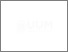UUM Electronic Theses and Dissertation
UUM ETD | Universiti Utara Malaysian Electronic Theses and Dissertation
FAQs | Feedback | Search Tips | Sitemap

# New alternative statistic for testing several independent samples of correlation matrices in high dimension data

Atiany, Tareq A.M. (2018) New alternative statistic for testing several independent samples of correlation matrices in high dimension data. PhD. thesis, Universiti Utara Malaysia.Preview
Text
DepositPermission_s95832.pdfPreview
Text
s95832_01.pdfPreview
Text
s95832_02.pdf

## Abstract

Jennrich Jennrich statistic is one of the existing statistics which is used for testing the equality of several independent samples of correlation matrices. The statistic is
gaining considerable importance in several areas of economics and financial markets. In these research areas, the number of variables, p, is usually larger than the sample size, n, which is known as high dimension data p > n. Subsequently, the estimation of correlation and covariance determinant will breakdown due to singularity problem. When this happens, Jennrich statistic is unable to function as the calculation involves the inversion of correlation matrix. Therefore, to resolve the aforementioned problem, this study develops an alternative statistic for testing several independent samples of correlation matrices in high dimension data. For this reason, the algebraic approach on the basis of vec operator, commutation matrix and Frobenius norm of
upper-off-diagonal elements are used to derive the new asymptotic distribution for the new alternative statistic, namely * Z statistic. Simulation study was conducted by considering different number of variables, sample sizes, and correlation shifts to evaluate the performance of the new statistic. In addition, real data on Asia Pacific currencies structure during the Tohoku earthquake were applied to validate the new * Z statistic. The power of the * Z statistic is compared with the existing Jennrich
statistic, and * T statistic through simulation study. As a result, the power of * Z statistic dominates the power of Jennrich statistic and * T statistic in all conditions, especially, when the shift in correlation matrix is at least 0.3 As a conclusion, the theoretical and simulation results are established and supported by desirable power of test. Meanwhile, investigation on real data indicates that the new alternative statistic can accommodate high dimension data.

Item Type: Thesis (PhD.) Sharif, Shamshuritawati 6889 Correlation matrix, Vec operator, Commutation matrix, Frobenius norm. Q Science > QA Mathematics > QA273-280 Probabilities. Mathematical statistics Awang Had Salleh Graduate School of Arts & Sciences 29 Nov 2018 06:57 09 Aug 2021 04:38 Awang Had Salleh Graduate School of Arts and Sciences Sharif, Shamshuritawati https://etd.uum.edu.my/id/eprint/6889